# Methods and formulas for the model summary in Random Forests® Classification

## Important variables

Minitab Statistical Software offers two methods to rank the importance of the variables.

### Permutation

The permutation method uses the out-of-bag data. For a given tree, j, in the analysis, classify the out-of-bag data with the tree. Repeat this classification for every tree in the forest. Then, compute the margin for each row that appears at least once in the out-of-bag data. The margin is the proportion of votes for the true class minus the maximum proportion of votes among the other classes. For example, suppose a row is in class A out of the available classes A, B, and C. The row appears in the out-of-bag data 100 times with the following classifications:
• A = 87
• B= 9
• C = 4

Then the margin for that row is 0.87 - 0.09 = 0.78.

The average out-of-bag margin is the average margin for all of the rows of data.

To determine the importance of the variable, randomly permute the values of a variable, xm through the out-of-bag data. Leave the response values and the other predictor values the same. Then, use the same steps to calculate the average margin for the permuted data,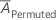.

The importance for variable xm comes from the difference of the two averages:

where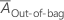is the average margin before the permutation. Minitab rounds values smaller than 10–7 to 0.

Repeat this process for every variable in the analysis. The variable with the highest importance is the most important variable. The relative variable importance scores are scaled by the importance of the most important variable:

### Gini

Any classification tree is a collection of splits. Each split provides improvement to the tree.

The following formula gives the improvement at a single node:

The improvement for a single tree is the sum of the squared improvements for the individual nodes:

where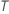is the number of nodes that split and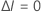for any nodewhere the variable of interest is not the splitter.

The improvement for an entire forest is the sum of the squared importances across all the trees in the forest:

Where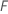is the number of trees in the forest and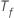is the number of nodes that split in tree.

The calculation of node impurity is similar to the Gini method. For details on the Gini method, go to Node splitting methods in CART® Classification.

The variable with the highest importance is the most important variable. The relative variable importance scores are scaled by the importance of the most important variable:

## Average –loglikelihood

Minitab calculates the average of the negative log-likelihood value when the response is binary. The calculations depend on the validation method.

### Out-of-bag data

The calculation uses the out-of-bag samples from every tree in the forest. Because of the nature of out-of-bag samples, expect to use different combinations of trees to find the contribution to the log-likelihood for each row in the data.

For a given tree in the forest, a class vote for a row in the out-of-bag data is the predicted class for the row from the single tree. The predicted class for a row in out-of-bag data is the class with the highest vote across all trees in the forest. The predicted class probability for a row in the out-of-bag data is the ratio of the number of votes for the class and the total votes for the row. The likelihood calculations follow from these probabilities:

where

and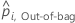is the calculated event probability for row i in the out-of-bag data.

### Notation for out-of-bag data

TermDescription
nOut-of-bagnumber of rows that are out-of-bag at least once
yi, Out-of-bagbinary response value of case i in the out-of-bag data. yi, Out-of-bag = 1 for event class, and 0 otherwise.

### Test set

For a given tree in the forest, a class vote for a row in the test set is the predicted class for the row from the single tree. The predicted class for a row in test set is the class with the highest vote across all trees in the forest. The predicted class probability for a row in the test set is the ratio of the number of votes for the class and the total votes for the row. The likelihood calculations follow from these probabilities:

where

### Notation for test set

TermDescription
nTestsample size of the test set
yi, Testbinary response value of case i in the test set. yi, k = 1 for event class, and 0 otherwise.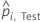predicted event probability for case i in the test set

## Area under ROC curve

The Model Summary table includes the area under the ROC curve when the response is binary. The ROC curve plots the true positive rate (TPR), also known as power, on the y-axis, and the false positive rate (FPR), also known as type 1 error, on the x-axis. The area under the ROC curve values typically range from 0.5 to 1.

### Formula

The area under the curve is a summation of areas of trapezoids:

where k is the number of distinct event probabilities and (x0, y0) is the point (0, 0).

To compute the area for a curve from out-of-bag data or a test set, use the points from the corresponding curve.

### Notation

TermDescription
TPR true positive rate
FPR false positive rate
TPtrue positive, events that were correctly assessed
FNfalse negative, events that were incorrectly assessed
P number of actual positive events
FPfalse positive, nonevents that were incorrectly assessed
N number of actual negative events
FNRfalse negative rate
TNRtrue negative rate

### Example

For example, suppose your results have 4 distinct fitted values with the following coordinates on the ROC curve:
x (false positive rate) y (true positive rate)
0.0923 0.3051
0.4154 0.7288
0.7538 0.9322
1 1
Then the area under the ROC curve is given by the following calculation: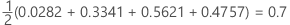## 95% CI for the area under the ROC curve

Minitab calculates a confidence interval for the area under the Receiver Operating Characteristic curve when the response is binary.

The following interval gives the upper and lower bounds for the confidence interval:

The computation of the standard error of the area under the ROC curve (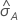) comes from Salford Predictive Modeler®. For general information about estimation of the variance of the area under the ROC curve, see the following references:

Engelmann, B. (2011). Measures of a ratings discriminative power: Applications and limitations. In B. Engelmann & R. Rauhmeier (Eds.), The Basel II Risk Parameters: Estimation, Validation, Stress Testing - With Applications to Loan Risk Management (2nd ed.) Heidelberg; New York: Springer. doi:10.1007/978-3-642-16114-8

Cortes, C. and Mohri, M. (2005). Confidence intervals for the area under the ROC curve. Advances in neural information processing systems, 305-312.

Feng, D., Cortese, G., & Baumgartner, R. (2017). A comparison of confidence/credible interval methods for the area under the ROC curve for continuous diagnostic tests with small sample size. Statistical Methods in Medical Research, 26(6), 2603-2621. doi:10.1177/0962280215602040

### Notation

TermDescription
Aarea under the ROC curve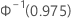0.975 percentile of the standard normal distribution

## Lift

Minitab displays lift in the model summary table when the response is binary. The lift in the model summary table is the cumulative lift for 10% of the data.

To see general calculations for cumulative lift, go to Methods and formulas for the cumulative lift chart for Random Forests® Classification.

## Misclassification rate

The following equation gives the misclassification rate:

The misclassed count is the number of rows in the out-of-bag data where their predicted classes are different from their true classes. Total count is the total number of rows in the out-of-bag data.

For validation with a test data set, the misclassed count is the sum of misclassifications in the test set. Total count is the number of rows in the test data set.